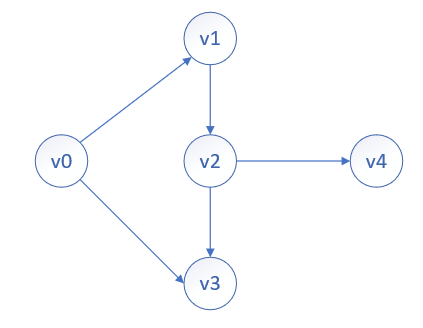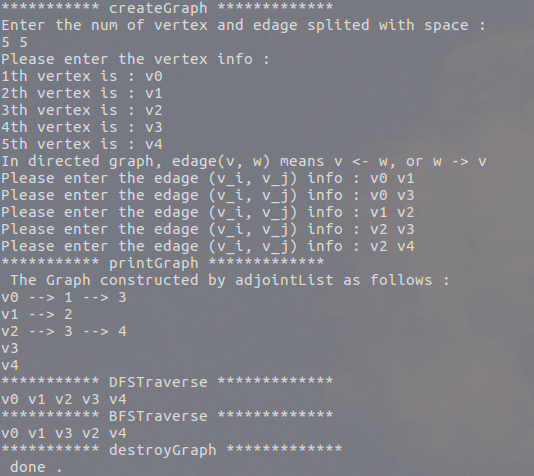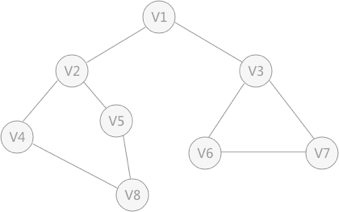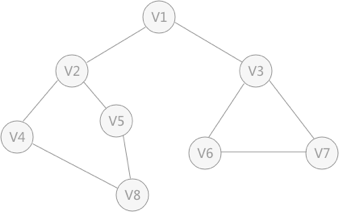• 在上一篇无向图之广度优先搜索和深度优先探索中简单介绍了图的基本概念、图的表示方法以及最短路径问题，本篇通过原有基础改变无向图为有向图，则原无向图为该有向图的基础图。改变无向图为有向图的基本思路为：创建...
        在上一篇无向图之广度优先搜索和深度优先探索中简单介绍了图的基本概念、图的表示方法以及最短路径问题，本篇通过原有基础改变无向图为有向图，则原无向图为该有向图的基础图。改变无向图为有向图的基本思路为：创建边时，不一定存在边，其中指顶点指向顶点​​​​的边【注不同的定义方式与编码有关，只要能反映图信息即可】。

因其更改很方便，这里直接给出代码，详细分析与无向图之广度优先搜索和深度优先探索基本一致。

创建如下有向图，则广度优先顺序为：，深度优先顺序为：。实例：基于有向图的广度优先搜索和深度优先搜索

//main.cpp
#include<iostream>
#include<vector>
#include<queue>
#include<string>

using namespace std;
const int N = 10;
vector<bool> visited(N);

struct EdageNode{
int adjointVertex;
EdageNode *next;

//EdageNode constructor
EdageNode(int a = 0, EdageNode *n = NULL):adjointVertex(a), next(n){

};
};

struct AdjointList{
string data;
EdageNode *firstEdage;
};

struct Graph{
int numVertex;
int numEdge;
vector<AdjointList> adjointList;

//Graph constructor
Graph(int n = 10):adjointList(n){
};
};

//create Graph g
void createGraph(Graph &g);

//get value's position in g
int getPosition(Graph g, string value);

//print the graph
void print(Graph g);

//Depth First Search for Graph g with index i
void DFS(Graph g, int index);

//Depth First Search Traverse
void DFSTraverse(Graph g);

//Breadth First Search Traverse
void BFSTraverse(Graph g);

//destroy Graph g
void destroyGraph(Graph &g);

int main(){
Graph g;
cout << "*********** createGraph *************" << endl;
createGraph(g);
cout << "*********** printGraph *************" << endl;
print(g);
cout << "*********** DFSTraverse *************" << endl;
DFSTraverse(g);
cout << "*********** BFSTraverse *************" << endl;
BFSTraverse(g);
cout << "*********** destroyGraph *************" << endl;
destroyGraph(g);
cout << " done ." << endl;
return 0;
}

void createGraph(Graph &g){
cout << "Enter the num of vertex and edage splited with space : " << endl;
cin >> g.numVertex >> g.numEdge;
cout << "Please enter the vertex info : " << endl;
for(int i = 0; i < g.numVertex; i++){
cout << i + 1 << "th vertex is : ";
cin >> g.adjointList[i].data;
g.adjointList[i].firstEdage = NULL;
}
int pos1, pos2;
string data1, data2;
EdageNode *node;
EdageNode *temp;
cout << "In directed graph, edage(v, w) means v -> w" << endl;
for(int i = 0; i < g.numEdge; i++){
cout << "Please enter the edage (v_i, v_j) info : ";
cin >> data1 >> data2;
pos1 = getPosition(g, data1);
pos2 = getPosition(g, data2);
//data1's firstEdage is NULL or not
if(g.adjointList[pos1].firstEdage == NULL){		//yes, connect data2 to data1 rear
node = new EdageNode(pos2, NULL);
g.adjointList[pos1].firstEdage = node;
}else{											//no, connect data2 to data1 until data1's next is NULL
temp = g.adjointList[pos1].firstEdage;
while(temp -> next != NULL){
temp = temp -> next;
}
node = new EdageNode(pos2, NULL);
temp -> next = node;
}
}
}

int getPosition(Graph g, string value){
for(int i = 0; i < g.numVertex; i++){
if(g.adjointList[i].data == value){
return i;
}
}
return -1;
}

void print(Graph g){
cout << " The Graph constructed by adjointList as follows : " << endl;
for(int i = 0; i < g.numVertex; i++){
cout << g.adjointList[i].data;
//access each data and find its next data
EdageNode *node = g.adjointList[i].firstEdage;
while(node){
cout << " --> " << node -> adjointVertex;
node = node -> next;
}
cout << endl;
}
}

void DFS(Graph g, int index){
EdageNode *node;
visited[index] = true;
cout << g.adjointList[index].data << " ";
node = g.adjointList[index].firstEdage;
//access data until the node is NULL
while(node){
if(!visited[node -> adjointVertex]){
DFS(g, node -> adjointVertex);
}
node = node -> next;
}
}

void DFSTraverse(Graph g){
for(int i = 0; i < g.numVertex; i++){
visited[i] = false;
}
for(int i = 0; i < g.numVertex; i++){
if(!visited[i]){
DFS(g, i);
}
}
cout << endl;
}

void BFSTraverse(Graph g){
EdageNode *node;
queue<int> bfsQueue;
vector<bool> visited(g.numVertex);
for(int i = 0; i < g.numVertex; i++){
visited[i] = false;
}
for(int i = 0; i < g.numVertex; i++){
if(!visited[i]){
visited[i] = true;
cout << g.adjointList[i].data << " ";
bfsQueue.push(i);
while(!bfsQueue.empty()){
int count = bfsQueue.front();
bfsQueue.pop();
node = g.adjointList[i].firstEdage;
//access firstEdage until node is NULL
while(node){
if(!visited[node -> adjointVertex]){
visited[node -> adjointVertex] = true;
cout << g.adjointList[node -> adjointVertex].data << " ";
bfsQueue.push(node -> adjointVertex);
}
node = node -> next;
}
}
}
}
cout << endl;
}

void destroyGraph(Graph &g){
EdageNode *temp = NULL;
for(int i = 0; i < g.numVertex; i++){
temp = g.adjointList[i].firstEdage;
while(temp){
EdageNode *node = temp;
temp = temp -> next;
delete node;
}
g.adjointList[i].firstEdage = NULL;
}
}


实验结果：practice makes perfect !

展开全文有向图
• 前边介绍了有关图的 4 种存储方式，本...常用的遍历方式有两种：深度优先搜索和广度优先搜索深度优先搜索（简称“深搜”或DFS） 图 1 无向图 深度优先搜索的过程类似于树的先序遍历，首先从例子中体会深度...
前边介绍了有关图的 4 种存储方式，本节介绍如何对存储的图中的顶点进行遍历。常用的遍历方式有两种：深度优先搜索和广度优先搜索。

深度优先搜索（简称“深搜”或DFS）图 1 无向图

深度优先搜索的过程类似于树的先序遍历，首先从例子中体会深度优先搜索。例如图 1 是一个无向图，采用深度优先算法遍历这个图的过程为：

首先任意找一个未被遍历过的顶点，例如从 V1 开始，由于 V1 率先访问过了，所以，需要标记 V1 的状态为访问过；
然后遍历 V1 的邻接点，例如访问 V2 ，并做标记，然后访问 V2 的邻接点，例如 V4 （做标记），然后 V8 ，然后 V5 ；
当继续遍历 V5 的邻接点时，根据之前做的标记显示，所有邻接点都被访问过了。此时，从 V5 回退到 V8 ，看 V8 是否有未被访问过的邻接点，如果没有，继续回退到 V4 ， V2 ， V1 ；
通过查看 V1 ，找到一个未被访问过的顶点 V3 ，继续遍历，然后访问 V3  邻接点 V6 ，然后 V7 ；
由于 V7 没有未被访问的邻接点，所有回退到 V6 ，继续回退至 V3 ，最后到达 V1 ，发现没有未被访问的；
最后一步需要判断是否所有顶点都被访问，如果还有没被访问的，以未被访问的顶点为第一个顶点，继续依照上边的方式进行遍历。

根据上边的过程，可以得到图 1 通过深度优先搜索获得的顶点的遍历次序为：

V1 -> V2 -> V4 -> V8 -> V5 -> V3 -> V6 -> V7

所谓深度优先搜索，是从图中的一个顶点出发，每次遍历当前访问顶点的临界点，一直到访问的顶点没有未被访问过的临界点为止。然后采用依次回退的方式，查看来的路上每一个顶点是否有其它未被访问的临界点。访问完成后，判断图中的顶点是否已经全部遍历完成，如果没有，以未访问的顶点为起始点，重复上述过程。

深度优先搜索是一个不断回溯的过程。

采用深度优先搜索算法遍历图的实现代码为：

#include <stdio.h>

#define MAX_VERtEX_NUM 20                   //顶点的最大个数
#define VRType int                          //表示顶点之间的关系的变量类型
#define InfoType char                       //存储弧或者边额外信息的指针变量类型
#define VertexType int                      //图中顶点的数据类型

typedef enum{false,true}bool;               //定义bool型常量
bool visited[MAX_VERtEX_NUM];               //设置全局数组，记录标记顶点是否被访问过

typedef struct {
VRType adj;                             //对于无权图，用 1 或 0 表示是否相邻；对于带权图，直接为权值。
InfoType * info;                        //弧或边额外含有的信息指针
}ArcCell,AdjMatrix[MAX_VERtEX_NUM][MAX_VERtEX_NUM];

typedef struct {
VertexType vexs[MAX_VERtEX_NUM];        //存储图中顶点数据
AdjMatrix arcs;                         //二维数组，记录顶点之间的关系
int vexnum,arcnum;                      //记录图的顶点数和弧（边）数
}MGraph;
//根据顶点本身数据，判断出顶点在二维数组中的位置
int LocateVex(MGraph * G,VertexType v){
int i=0;
//遍历一维数组，找到变量v
for (; i<G->vexnum; i++) {
if (G->vexs[i]==v) {
break;
}
}
//如果找不到，输出提示语句，返回-1
if (i>G->vexnum) {
printf("no such vertex.\n");
return -1;
}
return i;
}
//构造无向图
void CreateDN(MGraph *G){
scanf("%d,%d",&(G->vexnum),&(G->arcnum));
for (int i=0; i<G->vexnum; i++) {
scanf("%d",&(G->vexs[i]));
}
for (int i=0; i<G->vexnum; i++) {
for (int j=0; j<G->vexnum; j++) {
G->arcs[i][j].adj=0;
G->arcs[i][j].info=NULL;
}
}
for (int i=0; i<G->arcnum; i++) {
int v1,v2;
scanf("%d,%d",&v1,&v2);
int n=LocateVex(G, v1);
int m=LocateVex(G, v2);
if (m==-1 ||n==-1) {
printf("no this vertex\n");
return;
}
G->arcs[n][m].adj=1;
G->arcs[m][n].adj=1;//无向图的二阶矩阵沿主对角线对称
}
}

int FirstAdjVex(MGraph G,int v)
{
//查找与数组下标为v的顶点之间有边的顶点，返回它在数组中的下标
for(int i = 0; i<G.vexnum; i++){
if( G.arcs[v][i].adj ){
return i;
}
}
return -1;
}
int NextAdjVex(MGraph G,int v,int w)
{
//从前一个访问位置w的下一个位置开始，查找之间有边的顶点
for(int i = w+1; i<G.vexnum; i++){
if(G.arcs[v][i].adj){
return i;
}
}
return -1;
}
void visitVex(MGraph G, int v){
printf("%d ",G.vexs[v]);
}
void DFS(MGraph G,int v){
visited[v] = true;//标记为true
visitVex( G,  v); //访问第v 个顶点
//从该顶点的第一个边开始，一直到最后一个边，对处于边另一端的顶点调用DFS函数
for(int w = FirstAdjVex(G,v); w>=0; w = NextAdjVex(G,v,w)){
//如果该顶点的标记位false，证明未被访问，调用深度优先搜索函数
if(!visited[w]){
DFS(G,w);
}
}
}
//深度优先搜索
void DFSTraverse(MGraph G){//
int v;
//将用做标记的visit数组初始化为false
for( v = 0; v < G.vexnum; ++v){
visited[v] = false;
}
//对于每个标记为false的顶点调用深度优先搜索函数
for( v = 0; v < G.vexnum; v++){
//如果该顶点的标记位为false，则调用深度优先搜索函数
if(!visited[v]){
DFS( G, v);
}
}
}

int main() {
MGraph G;//建立一个图的变量
CreateDN(&G);//初始化图
DFSTraverse(G);//深度优先搜索图
return 0;
}

以图 1 为例，运行结果为：

8,9
1
2
3
4
5
6
7
8
1,2
2,4
2,5
4,8
5,8
1,3
3,6
6,7
7,3
1 2 4 8 5 3 6 7

广度优先搜索

广度优先搜索类似于树的层次遍历。从图中的某一顶点出发，遍历每一个顶点时，依次遍历其所有的邻接点，然后再从这些邻接点出发，同样依次访问它们的邻接点。按照此过程，直到图中所有被访问过的顶点的邻接点都被访问到。
最后还需要做的操作就是查看图中是否存在尚未被访问的顶点，若有，则以该顶点为起始点，重复上述遍历的过程。
还拿图 1 中的无向图为例，假设 V1 作为起始点，遍历其所有的邻接点 V2 和 V3 ，以 V2 为起始点，访问邻接点 V4 和 V5 ，以 V3 为起始点，访问邻接点 V6 、 V7 ，以 V4 为起始点访问 V8 ，以 V5 为起始点，由于 V5 所有的起始点已经全部被访问，所有直接略过， V6 和 V7 也是如此。
以 V1 为起始点的遍历过程结束后，判断图中是否还有未被访问的点，由于图 1 中没有了，所以整个图遍历结束。遍历顶点的顺序为：

V1 -> V2 -> v3 -> V4 -> V5 -> V6 -> V7 -> V8

广度优先搜索的实现需要借助队列这一特殊数据结构，实现代码为：

#include <stdio.h>
#include <stdlib.h>
#define MAX_VERtEX_NUM 20                   //顶点的最大个数
#define VRType int                          //表示顶点之间的关系的变量类型
#define InfoType char                       //存储弧或者边额外信息的指针变量类型
#define VertexType int                      //图中顶点的数据类型
typedef enum{false,true}bool;               //定义bool型常量
bool visited[MAX_VERtEX_NUM];               //设置全局数组，记录标记顶点是否被访问过
typedef struct Queue{
VertexType data;
struct Queue * next;
}Queue;
typedef struct {
VRType adj;                             //对于无权图，用 1 或 0 表示是否相邻；对于带权图，直接为权值。
InfoType * info;                        //弧或边额外含有的信息指针
}ArcCell,AdjMatrix[MAX_VERtEX_NUM][MAX_VERtEX_NUM];

typedef struct {
VertexType vexs[MAX_VERtEX_NUM];        //存储图中顶点数据
AdjMatrix arcs;                         //二维数组，记录顶点之间的关系
int vexnum,arcnum;                      //记录图的顶点数和弧（边）数
}MGraph;
//根据顶点本身数据，判断出顶点在二维数组中的位置
int LocateVex(MGraph * G,VertexType v){
int i=0;
//遍历一维数组，找到变量v
for (; i<G->vexnum; i++) {
if (G->vexs[i]==v) {
break;
}
}
//如果找不到，输出提示语句，返回-1
if (i>G->vexnum) {
printf("no such vertex.\n");
return -1;
}
return i;
}
//构造无向图
void CreateDN(MGraph *G){
scanf("%d,%d",&(G->vexnum),&(G->arcnum));
for (int i=0; i<G->vexnum; i++) {
scanf("%d",&(G->vexs[i]));
}
for (int i=0; i<G->vexnum; i++) {
for (int j=0; j<G->vexnum; j++) {
G->arcs[i][j].adj=0;
G->arcs[i][j].info=NULL;
}
}
for (int i=0; i<G->arcnum; i++) {
int v1,v2;
scanf("%d,%d",&v1,&v2);
int n=LocateVex(G, v1);
int m=LocateVex(G, v2);
if (m==-1 ||n==-1) {
printf("no this vertex\n");
return;
}
G->arcs[n][m].adj=1;
G->arcs[m][n].adj=1;//无向图的二阶矩阵沿主对角线对称
}
}

int FirstAdjVex(MGraph G,int v)
{
//查找与数组下标为v的顶点之间有边的顶点，返回它在数组中的下标
for(int i = 0; i<G.vexnum; i++){
if( G.arcs[v][i].adj ){
return i;
}
}
return -1;
}
int NextAdjVex(MGraph G,int v,int w)
{
//从前一个访问位置w的下一个位置开始，查找之间有边的顶点
for(int i = w+1; i<G.vexnum; i++){
if(G.arcs[v][i].adj){
return i;
}
}
return -1;
}
//操作顶点的函数
void visitVex(MGraph G, int v){
printf("%d ",G.vexs[v]);
}
//初始化队列
void InitQueue(Queue ** Q){
(*Q)=(Queue*)malloc(sizeof(Queue));
(*Q)->next=NULL;
}
//顶点元素v进队列
void EnQueue(Queue **Q,VertexType v){
Queue * element=(Queue*)malloc(sizeof(Queue));
element->data=v;
Queue * temp=(*Q);
while (temp->next!=NULL) {
temp=temp->next;
}
temp->next=element;
}
//队头元素出队列
void DeQueue(Queue **Q,int *u){
(*u)=(*Q)->next->data;
(*Q)->next=(*Q)->next->next;
}
//判断队列是否为空
bool QueueEmpty(Queue *Q){
if (Q->next==NULL) {
return true;
}
return false;
}
//广度优先搜索
void BFSTraverse(MGraph G){//
int v;
//将用做标记的visit数组初始化为false
for( v = 0; v < G.vexnum; ++v){
visited[v] = false;
}
//对于每个标记为false的顶点调用深度优先搜索函数
Queue * Q;
InitQueue(&Q);
for( v = 0; v < G.vexnum; v++){
if(!visited[v]){
visited[v]=true;
visitVex(G, v);
EnQueue(&Q, G.vexs[v]);
while (!QueueEmpty(Q)) {
int u;
DeQueue(&Q, &u);
u=LocateVex(&G, u);
for (int w=FirstAdjVex(G, u); w>=0; w=NextAdjVex(G, u, w)) {
if (!visited[w]) {
visited[w]=true;
visitVex(G, w);
EnQueue(&Q, G.vexs[w]);
}
}
}
}
}
}
int main() {
MGraph G;//建立一个图的变量
CreateDN(&G);//初始化图
BFSTraverse(G);//广度优先搜索图
return 0;
}

例如，使用上述程序代码遍历图 1 中的无向图，运行结果为：

8,9
1
2
3
4
5
6
7
8
1,2
2,4
2,5
4,8
5,8
1,3
3,6
6,7
7,3
1 2 3 4 5 6 7 8

总结

本节介绍了两种遍历图的方式：深度优先搜索算法和广度优先搜索算法。深度优先搜索算法的实现运用的主要是回溯法，类似于树的先序遍历算法。广度优先搜索算法借助队列的先进先出的特点，类似于树的层次遍历。

数据结构教程已经过作者多次打磨和润色，现已初具规模。教程分为入门教程（免费）和高级课程（收费），有想深入学习数据结构，购买高级课程的读者，可联系作者（QQ：834937624（备注信息：购买教程）），或登录数据结构网站获取。

展开全文DFS和BFS
• 常用的遍历方式有两种：深度优先搜索和广度优先搜索。 深度优先搜索（简称“深搜”或DFS） 图 1 无向图 深度优先搜索的过程类似于树的先序遍历，首先从例子中体会深度优先搜索。例如图 1 是一个无向图，采用深度...

前边介绍了有关图的 4 种存储方式，本节介绍如何对存储的图中的顶点进行遍历。常用的遍历方式有两种：深度优先搜索和广度优先搜索。

深度优先搜索（简称“深搜”或DFS）图 1 无向图

深度优先搜索的过程类似于树的先序遍历，首先从例子中体会深度优先搜索。例如图 1 是一个无向图，采用深度优先算法遍历这个图的过程为：

首先任意找一个未被遍历过的顶点，例如从 V1 开始，由于 V1 率先访问过了，所以，需要标记 V1 的状态为访问过；
然后遍历 V1 的邻接点，例如访问 V2 ，并做标记，然后访问 V2 的邻接点，例如 V4 （做标记），然后 V8 ，然后 V5 ；
当继续遍历 V5 的邻接点时，根据之前做的标记显示，所有邻接点都被访问过了。此时，从 V5 回退到 V8 ，看 V8 是否有未被访问过的邻接点，如果没有，继续回退到 V4 ， V2 ， V1 ；
通过查看 V1 ，找到一个未被访问过的顶点 V3 ，继续遍历，然后访问 V3  邻接点 V6 ，然后 V7 ；
由于 V7 没有未被访问的邻接点，所有回退到 V6 ，继续回退至 V3 ，最后到达 V1 ，发现没有未被访问的；
最后一步需要判断是否所有顶点都被访问，如果还有没被访问的，以未被访问的顶点为第一个顶点，继续依照上边的方式进行遍历。

根据上边的过程，可以得到图 1 通过深度优先搜索获得的顶点的遍历次序为：V1 -> V2 -> V4 -> V8 -> V5 -> V3 -> V6 -> V7
所谓深度优先搜索，是从图中的一个顶点出发，每次遍历当前访问顶点的邻接点，一直到访问的顶点没有未被访问过的邻接点为止。然后采用依次回退的方式，查看来的路上每一个顶点是否有其它未被访问的邻接点。访问完成后，判断图中的顶点是否已经全部遍历完成，如果没有，以未访问的顶点为起始点，重复上述过程。

深度优先搜索是一个不断回溯的过程。

采用深度优先搜索算法遍历图的实现代码为（邻接矩阵+无向图（有向图也是如此））：
#include <stdio.h>

#define MAX_VERtEX_NUM 20                   //顶点的最大个数
#define VRType int                          //表示顶点之间的关系的变量类型
#define InfoType char                       //存储弧或者边额外信息的指针变量类型
#define VertexType int                      //图中顶点的数据类型

typedef enum{false,true}bool;               //定义bool型常量
bool visited[MAX_VERtEX_NUM];               //设置全局数组，记录标记顶点是否被访问过

typedef struct {
VRType adj;                             //对于无权图，用 1 或 0 表示是否相邻；对于带权图，直接为权值。
InfoType * info;                        //弧或边额外含有的信息指针
}ArcCell,AdjMatrix[MAX_VERtEX_NUM][MAX_VERtEX_NUM];

typedef struct {
VertexType vexs[MAX_VERtEX_NUM];        //存储图中顶点数据
AdjMatrix arcs;                         //二维数组，记录顶点之间的关系
int vexnum,arcnum;                      //记录图的顶点数和弧（边）数
}MGraph;

//根据顶点本身数据，判断出顶点在二维数组中的位置
int LocateVex(MGraph * G,VertexType v){
int i=0;
//遍历一维数组，找到变量v
for (; i<G->vexnum; i++) {
if (G->vexs[i]==v) {
break;
}
}
//如果找不到，输出提示语句，返回-1
if (i>G->vexnum) {
printf("no such vertex.\n");
return -1;
}
return i;
}

//构造无向图
void CreateDN(MGraph *G){
scanf("%d,%d",&(G->vexnum),&(G->arcnum));
for (int i=0; i<G->vexnum; i++) {
scanf("%d",&(G->vexs[i]));
}
for (int i=0; i<G->vexnum; i++) {
for (int j=0; j<G->vexnum; j++) {
G->arcs[i][j].adj=0;
G->arcs[i][j].info=NULL;
}
}
for (int i=0; i<G->arcnum; i++) {
int v1,v2;
scanf("%d,%d",&v1,&v2);
int n=LocateVex(G, v1);
int m=LocateVex(G, v2);
if (m==-1 ||n==-1) {
printf("no this vertex\n");
return;
}
G->arcs[n][m].adj=1;
G->arcs[m][n].adj=1;//无向图的二阶矩阵沿主对角线对称
}
}

int FirstAdjVex(MGraph G,int v)
{
//查找与数组下标为v的顶点之间有边的顶点，返回它在数组中的下标
for(int i = 0; i<G.vexnum; i++){
if( G.arcs[v][i].adj ){
return i;
}
}
return -1;
}

int NextAdjVex(MGraph G,int v,int w)
{
//从前一个访问位置w的下一个位置开始，查找之间有边的顶点
for(int i = w+1; i<G.vexnum; i++){
if(G.arcs[v][i].adj){
return i;
}
}
return -1;
}

void visitVex(MGraph G, int v){
printf("%d ",G.vexs[v]);
}

void DFS(MGraph G,int v){
visited[v] = true;//标记为true
visitVex( G,  v); //访问第v 个顶点
//从该顶点的第一个边开始，一直到最后一个边，对处于边另一端的顶点调用DFS函数
for(int w = FirstAdjVex(G,v); w>=0; w = NextAdjVex(G,v,w)){
//如果该顶点的标记位false，证明未被访问，调用深度优先搜索函数
if(!visited[w]){
DFS(G,w);
}
}
}

//深度优先搜索
void DFSTraverse(MGraph G){//
int v;
//将用做标记的visit数组初始化为false
for( v = 0; v < G.vexnum; ++v){
visited[v] = false;
}
//对于每个标记为false的顶点调用深度优先搜索函数
for( v = 0; v < G.vexnum; v++){
//如果该顶点的标记位为false，则调用深度优先搜索函数
if(!visited[v]){
DFS( G, v);
}
}
}

int main() {
MGraph G;//建立一个图的变量
CreateDN(&G);//初始化图
DFSTraverse(G);//深度优先搜索图
return 0;
}

以图 1 为例，运行结果为：
8,9
1
2
3
4
5
6
7
8
1,2
2,4
2,5
4,8
5,8
1,3
3,6
6,7
7,3
1 2 4 8 5 3 6 7

广度优先搜索

广度优先搜索类似于树的层次遍历。从图中的某一顶点出发，遍历每一个顶点时，依次遍历其所有的邻接点，然后再从这些邻接点出发，同样依次访问它们的邻接点。按照此过程，直到图中所有被访问过的顶点的邻接点都被访问到。
最后还需要做的操作就是查看图中是否存在尚未被访问的顶点，若有，则以该顶点为起始点，重复上述遍历的过程。
还拿图 1 中的无向图为例：

假设 V1 作为起始点，遍历其所有的邻接点 V2 和 V3；
然后以 V2 为起始点，访问邻接点 V4 和 V5 ；
然后以 V3 为起始点，访问邻接点 V6 、 V7 ；
然后以 V4 为起始点访问 V8 ；
然后以 V5 为起始点，由于 V5 所有的起始点已经全部被访问，所有直接略过；
然后V6 和 V7 也是如此。
最后，以 V1 为起始点的遍历过程结束后，还需判断图中是否还有未被访问的点，由于图 1 中没有了，所以整个图遍历结束。如果还有没被访问的，以未被访问的顶点为第一个顶点，继续依照上边的方式进行遍历。

根据上边的过程，可以得到图 1 通过广度优先搜索获得的顶点的遍历次序为：V1 -> V2 -> v3 -> V4 -> V5 -> V6 -> V7 -> V8
广度优先搜索的实现需要借助队列这一特殊数据结构，实现代码为（邻接矩阵+无向图（有向图也是如此））：：
#include <stdio.h>
#include <stdlib.h>
#define MAX_VERtEX_NUM 20                   //顶点的最大个数
#define VRType int                          //表示顶点之间的关系的变量类型
#define InfoType char                       //存储弧或者边额外信息的指针变量类型
#define VertexType int                      //图中顶点的数据类型
typedef enum{false,true}bool;               //定义bool型常量
bool visited[MAX_VERtEX_NUM];               //设置全局数组，记录标记顶点是否被访问过

typedef struct Queue{
VertexType data;
struct Queue * next;
}Queue;

typedef struct {
VRType adj;                             //对于无权图，用 1 或 0 表示是否相邻；对于带权图，直接为权值。
InfoType * info;                        //弧或边额外含有的信息指针
}ArcCell,AdjMatrix[MAX_VERtEX_NUM][MAX_VERtEX_NUM];

typedef struct {
VertexType vexs[MAX_VERtEX_NUM];        //存储图中顶点数据
AdjMatrix arcs;                         //二维数组，记录顶点之间的关系
int vexnum,arcnum;                      //记录图的顶点数和弧（边）数
}MGraph;

//根据顶点本身数据，判断出顶点在二维数组中的位置
int LocateVex(MGraph * G,VertexType v){
int i=0;
//遍历一维数组，找到变量v
for (; i<G->vexnum; i++) {
if (G->vexs[i]==v) {
break;
}
}
//如果找不到，输出提示语句，返回-1
if (i>G->vexnum) {
printf("no such vertex.\n");
return -1;
}
return i;
}

//构造无向图
void CreateDN(MGraph *G){
scanf("%d,%d",&(G->vexnum),&(G->arcnum));
for (int i=0; i<G->vexnum; i++) {
scanf("%d",&(G->vexs[i]));
}
for (int i=0; i<G->vexnum; i++) {
for (int j=0; j<G->vexnum; j++) {
G->arcs[i][j].adj=0;
G->arcs[i][j].info=NULL;
}
}
for (int i=0; i<G->arcnum; i++) {
int v1,v2;
scanf("%d,%d",&v1,&v2);
int n=LocateVex(G, v1);
int m=LocateVex(G, v2);
if (m==-1 ||n==-1) {
printf("no this vertex\n");
return;
}
G->arcs[n][m].adj=1;
G->arcs[m][n].adj=1;//无向图的二阶矩阵沿主对角线对称
}
}

int FirstAdjVex(MGraph G,int v)
{
//查找与数组下标为v的顶点之间有边的顶点，返回它在数组中的下标
for(int i = 0; i<G.vexnum; i++){
if( G.arcs[v][i].adj ){
return i;
}
}
return -1;
}

int NextAdjVex(MGraph G,int v,int w)
{
//从前一个访问位置w的下一个位置开始，查找之间有边的顶点
for(int i = w+1; i<G.vexnum; i++){
if(G.arcs[v][i].adj){
return i;
}
}
return -1;
}

//操作顶点的函数
void visitVex(MGraph G, int v){
printf("%d ",G.vexs[v]);
}

//初始化队列
void InitQueue(Queue ** Q){
(*Q)=(Queue*)malloc(sizeof(Queue));
(*Q)->next=NULL;
}

//顶点元素v进队列
void EnQueue(Queue **Q,VertexType v){
Queue * element=(Queue*)malloc(sizeof(Queue));
element->data=v;
Queue * temp=(*Q);
while (temp->next!=NULL) {
temp=temp->next;
}
temp->next=element;
}

//队头元素出队列
void DeQueue(Queue **Q,int *u){
(*u)=(*Q)->next->data;
(*Q)->next=(*Q)->next->next;
}

//判断队列是否为空
bool QueueEmpty(Queue *Q){
if (Q->next==NULL) {
return true;
}
return false;
}

//广度优先搜索
void BFSTraverse(MGraph G){//
int v;
//将用做标记的visit数组初始化为false
for( v = 0; v < G.vexnum; ++v){
visited[v] = false;
}
//对于每个标记为false的顶点调用深度优先搜索函数
Queue * Q;
InitQueue(&Q);
for( v = 0; v < G.vexnum; v++){
if(!visited[v]){
visited[v]=true;
visitVex(G, v);
EnQueue(&Q, G.vexs[v]);
while (!QueueEmpty(Q)) {
int u;
DeQueue(&Q, &u);
u=LocateVex(&G, u);
for (int w=FirstAdjVex(G, u); w>=0; w=NextAdjVex(G, u, w)) {
if (!visited[w]) {
visited[w]=true;
visitVex(G, w);
EnQueue(&Q, G.vexs[w]);
}
}
}
}
}
}

int main() {
MGraph G;//建立一个图的变量
CreateDN(&G);//初始化图
BFSTraverse(G);//广度优先搜索图
return 0;
}

例如，使用上述程序代码遍历图 1 中的无向图，运行结果为：
8,9
1
2
3
4
5
6
7
8
1,2
2,4
2,5
4,8
5,8
1,3
3,6
6,7
7,3
1 2 3 4 5 6 7 8

总结

本节介绍了两种遍历图的方式：深度优先搜索算法和广度优先搜索算法。深度优先搜索算法的实现运用的主要是回溯法，类似于树的先序遍历算法。广度优先搜索算法借助队列的先进先出的特点，类似于树的层次遍历。


展开全文DFS BFS 数据结构 算法
• 路径查找器 Artemas J.... 使用 PathFinder，您可以可视化广度优先搜索深度优先搜索和 A* 寻路算法如何在您自己的自定义绘制图形上运行。 供计算机科学专业的学生使用。 适用于 iOS、ipadOS 和 macOS。
• 首先先看下面这个有关迷宫的问题引入思考。 密室逃脱 Description 每天刷题太枯燥了，为了放松一下自己，你，zjxhhy约好了周末一起去玩密室逃脱。一般的密室逃脱对你们没有什么吸引力了，所以你们找到了一个更...
DFS
首先先看下面这个有关迷宫的问题引入思考。
密室逃脱
Description
每天刷题太枯燥了，为了放松一下自己，你，zjx和hhy约好了周末一起去玩密室逃脱。一般的密室逃脱对你们没有什么吸引力了，所以你们找到了一个更好玩的密室逃脱。
我们可以把整个密室看成一个 n * m 的网格图(包含很多房间)，其中每个房间都是一个1 * 1的单元格， 有一些房间有机关，进入后会失去游戏资格。我们将没有机关的房间用0表示，有机关的房间用1表示。
迷宫的规则是开局的时候zjx和hhy被随机放到一个房间里（保证被放房间是没有机关的），单位时间内他们可以选择进入到相邻的房间（上下左右），也可以选择不动。两人相遇即可通过游戏，越短时间通过游戏获得的奖品越好，所以你们要在最短的时间通过游戏，触碰机关失去游戏资格不会获得奖品。你在密室外指挥他们从自己的起点出发使他们相遇（你可以看到迷宫的地图并且你们之间可以联系），现在请你计算出他们两个从出发点到相遇需要的最短时间。
Input
第一行两个整数n, m表示网格图的大小。
第二行四个整数x1, y1, x2, y2分别表示zjx和hhy的起点横纵坐标。
接下来一个矩阵：n行，每行m个数（只含0和1），表示地图。
1 <= n, m <= 100,   1 <= x1, x2 <= n,  1 <= y1, y2 <= m
Output
一行，一个整数，表示zjx和hhy相遇的最短时间。
思路：
一看题目很多的字，但是其实你耐心读下去，提取信息会发现很简单，就是两个憨憨一起走一个迷宫，俩人都有自己的初始位置，他们同时走，每走一步都消耗一样的单位时间。然后问他们俩相遇的最短时间。
我们简化问题，两个人同时走让你不好思考，那你就把问题转化为一个人不动，然后另一个人来找这个人的最短路max_sum径是多少（因为走单位格移动的时间是单位时间，换成求路径更方便你思考），之后这个max_sum再除以2就是他们俩同时走的时间了。（当然，你思考一下，直接除以2是完全正确的吗？）
当然不是的了，因为两个人之间的最优路线的路径是奇数的话，那么势必有一个人要多走一步，判断一下加一即可，是偶数的时候便直接除以2.
下面开始讲核心算法DFS/BFS.
BFS
相当于一个队列，队列没学好的可以看下队列的基本知识便可以更好理解BFS的运用。
首先是存图，可以用最简单的二维表（二维数组）的方式存一个图。循环嵌套存入地图，应该不用过多讲解了。之后是定义一个队列，这个队列你可以自己手动写一个，也可以直接用STL里的queue队列。接着是一个循环，每次都要处理队列头的位置，寻找出这个位置能走到的所有位置，把更新出来的位置入队。之后删掉表头。这样就可以实现“千军万马”地毯式搜索的效果了。
例如此时队列头的位置在start(2,1),

1
start

1

1

end

那么你下一步可以走的位置可以是(1,2),(2,3),(3,2)，(2,1)有障碍物不能走。

here

1
start
here

here

1

1

end

你可能会疑惑如何让他判断所有下一步能走的点，你需要定义一个位置数组，这里我习惯定义一个nest数组,nest={ {0,1},{1.0},{0,-1},{-1,0} }，也就是说循环一下就会每次依次默认向右，下，左，上，进行试探搜索。比方说startx=2,starty=1,那么下一步向右t，x=2+0=2，ty=1+1=2，（2，2）没有障碍物，可以走，把这个位置入队，你可以思考一下整个搜索的过程，如果地图没问题，最先搜索到的目的位置的那条线也就是最短距离，也就是你要找的答案。
（DFS和BFS的具体实践等有空再更，你可以试着直接写一下DFS/BFS试一试）
代码如下：
#include <iostream>
#include <stdio.h>
using namespace std;
int n,m,map={0},book={0};
struct node{
int x;
int y;
int step;
};
int nest={{0,1},{1,0},{-1,0},{0,-1}};
node queue;
int main()
{
int i,j,head,tail,a,b,tx,ty,x1,y1,x2,y2;
cin>>n>>m>>x1>>y1>>x2>>y2;
for(i=1;i<=n;i++)
{
for(j=1;j<=m;j++)
{
cin>>map[i][j];
}
}
head=1;
tail=1;
queue[tail].x=x1;
queue[tail].y=y1;
queue[tail].step=0;
book[x1][y1]=1;
tail++;
while(head!=tail)
{
for(i=0;i<4;i++)
{
tx=queue[head].x+nest[i];
ty=queue[head].y+nest[i];
if(tx>n||ty>m||tx<1||ty<1)
{
continue;
}
if(book[tx][ty]==0&&map[tx][ty]==0)
{
queue[tail].x=tx;
queue[tail].y=ty;
queue[tail].step=queue[head].step+1;
book[tx][ty]=1;
if(tx==x2&&ty==y2)
{
if(queue[tail].step%2==0)
{
cout<<queue[tail].step/2<<endl;
break;
}
else
cout<<queue[tail].step/2+1<<endl;
break;
}
tail++;
}
}
head++;
}
cout<<endl;
return 0;
}



展开全文dfs
• 本章的第一节中，介绍了有关生成树生成森林的有关知识，本节来解决对于给定的无向图，如何构建它们相对应的生成树或者生成森林。...当使用深度优先搜索算法时，假设 V1 作为遍历的起始点，涉及到的顶点...数据结构 算法
• 广度优先搜索将图分离为层，每层之间间隔一步，每个点被遍历的次序遍历该图的初始点的距离有关。 如改图例子1（凑活把树当图看吧），按照深度优先搜索从点1开始遍历： 1–>2–>4，无法继续搜索，回退一步...队列 算法 dfs bfs
• 在图中进行搜索是一个非常重要的话题，具体来说，又分为深度优先搜索（DFS，Depth First Search）和广度优先搜索（BFS, Breadth First Search）两种。其中，前者的理解难度要大于后者，而且在Leetcode解题中，前者...DFS
• 在树（或者图）中进行搜索是一个非常重要的话题，具体来说，又分为深度优先搜索（DFS，Depth First Search）和广度优先搜索（BFS, Breadth First Search）两种。其中，前者的理解难度要大于后者，而且在Leetcode解题...
• 接下来我们将介绍一下有关图的基本的遍历算法，BFS（广度优先搜索遍历 ）DFS（深度优先搜索遍历 ）这两种遍历方式。 这里我们就以无向图来做示例： 无向图G1 DFS（深度优先遍历） 深...
• 广度优先搜索（BFS） 深度优先搜索（DFS） 迭代加深DFS（ID-DFS） 贪婪的搜索 A *搜索 由于贪婪搜索和A *搜索是已知的搜索算法，因此还提供了三种启发式功能： 欧氏距离启发式 曼哈顿距离启发式JavaScript
• 人工智能 该存储库致力于从罗马特雷大学的人工智能课程中汲取灵感的各种测试和人工智能算法。 该项目是使用C＃开发的App Console（.NET Core）。 玩具问题 ... 广度优先搜索和深度优先搜索-在递归模式下C#
• 迷宫的随机生成路径搜索主要图的遍历有关，一般来说图的遍历主要有两种方式：1、深度优先遍历（DFS）2、广度优先遍历（BFS）两种遍历方式都很好理解，就说说深度优先遍历：深度优先遍历，顾名思义，就是尽可能往...
• 图以及DFS和BFS的实现 前提，目标和结果 前提: 完成本次作业，学生需要掌握如下知识 • 图的存储- 有关图的存储的数据结构 • 图的便利-有关深度优先搜索和广度优先搜索的知识DFS BFS
• 深度优先算法拓扑算法是很多算法的基础,只得深究:/// /// 广度搜索算法,图 /// public partial class GraphicSearchAlg { /// /// 深度优先搜索算法,这里只是计算深度. /// /// 图,基于...算法 class null
• 图的遍历算法有深度优先搜索算法和广度优先搜索算法。 深度优先搜索(Depth First Search–DFS)遍历类似树的先序遍历，是树的先序遍历的推广。 深度优先搜索算法思想： 设初始状态时图中的所有顶点未被访问，则： ⑴ ...
• 1．用邻接表作为图的存储结构建立一个图，并对此图分别进行深度优先搜索和广度优先搜索遍历（验证性内容）。 2．用邻接矩阵作为图的存储结构建立一个网，并构造该网的最小生成树（设计性内容）。 三、实验要求 1...数据结构
• 迷宫的随机生成路径搜索主要图的遍历有关，一般来说图的遍历主要有两种方式：1、深度优先遍历（DFS）2、广度优先遍历（BFS） 两种遍历方式都很好理解，就说说深度优先遍历： 深度优先遍历，顾名思义，就是尽可能...
• 目录 写在前面 1.图的建立和遍历 2.最短路 3.拓扑排序 4.生成树 5.连通块 6.二分图，网络流等 ...这篇文章主要总结部分NOIP的图论知识点以及一些NOIP真题。...主要就是深度优先搜索和广度优先搜索两种搜...
• 深度优先搜索Depth First Search-DFS 广度优先搜索(Breadth First Search-BFS) 它们对无向图和有向图都适用 许多有关图的算法如求图的连通性问题拓扑排序求关键路径等都是在深度优先搜索和广度优先搜索的基本方法上...
• 如果无向图G必须进行两次广度优先搜索才能访问其所有顶点， 则G一定有2个连通分量（T） 【分析】连通分量：一个连通图只有一个连通分量， 两个连通分量说明有两个不连通的子图 无论 广度优先还是深度优先 最终实现的...数据结构
• Leetcode算法 说明：该存储库仍在更新中！...广度优先搜索 动态编程 回溯 贪婪的 递归 特里 分而治之 数学 魔法 两指针/滑动窗口 慢指针快指针 其他设计 版权 仅供学习，请拒绝转载。 Github@dai
• 广度优先搜索 深度优先搜索 Dijkstra的搜索 贡献给StructLinks 我们很高兴 :grinning_face_with_big_eyes: 您想为我们的项目做出贡献。 我们欢迎您加入我们的社区。 请查看我们的GuideToContributing页面，以获取...
• 文章目录计算机组成原理一道题计算题存储器相关DMA中断方式程序查询...有关编译程序解释程序c语言中不同变量分配的在哪一个区域数据结构子串的计算关于快速排序简单选择排序直接插入排序哈希函数模式匹配...
• 总结：解决有关的问题的时候，一般用到的方法有两种：深度优先搜索（DFS）和广度优先搜索（BFS）。广度优先搜索实质上就是层次遍历，每遍历完一层的所有节点才开始遍历下一层节点；深度优先搜索则是按分支去遍历...LeetCode C++
• ## 实验六 图的遍历

千次阅读 多人点赞 2017-12-21 21:36:46
3．掌握图的深度优先搜索和广度优先搜索遍历的方法及其计算机的实现。 4．理解最小生成树的有关算法   二、实验内容 1．用邻接表作为图的存储结构建立一个图，并对此图分别进行深度优先搜索和广度优先搜索遍历...数据结构 算法 c语言
• 最近在做有关树的题目的时候，很多时候都会遇到要遍历树。这里就不再说关于二叉树的遍历了。具体可以参考一下另一篇博客二叉树。...树图的遍历方式都可以用深度优先搜索（DFS）和广度优先搜索（BFS）来实现...
• Python程序 这是我收集的Python程序。 有关python教程，请...广度优先搜索 深度优先搜索 在有向图中检测周期 在无向图中检测周期 拓扑排序 普里姆算法 剧本 创建多个文件夹 计数文件 获取文件大小 查找文件是否存在
• 5.2 深度优先和广度优先查找;DFS的伪代码1;DFS的伪代码2;深度优先举例;深度优先搜索的效率与图的表示有关 对邻接矩阵表示的图 遍历的效率为 ? V ?2) ? 对邻接链表表示的图 遍历的效率为 ? V ?+? E ) ;2广度优先查找 ...